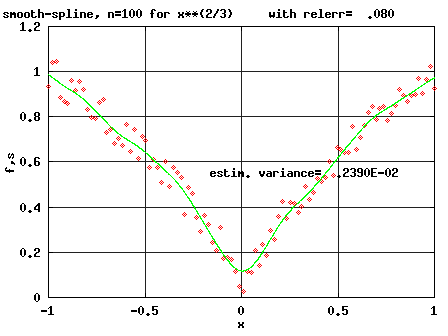## Approximation### The Problem: given data (x,f(x)) for x in a set X

a function g(x,P) , and a norm ||.|| on X
find parameters P such that
||f(.)-g(.,P)||is minimal with respect to P

For approximations to special functions search the netlib-libraries a, amos, cephes, elefunt, specfunc and the file netlib/toms/757 .
Here a list of some representative approximation algorithms is given. There are many more possibilities, e.g. using interpolation by splines, by splines under tension, by radial basis functions .... For more information use the search facility of netlib

### HOTGAMS

 APPROX/EXCH best polynomial approximation to a discrete one-dimensional data set in the Chebyshev (minimax) sense L1GNR generating test problems for discrete linear l1-approximation CDFCOR rational approximation to a finite set of data f90 version COCA Complex linear Chebyshev Approximation (Matlab) l2appr construct least squares approximation of B-splines to given data l2main driver for l2appr.f titan sample data for l2appr.f cubgcv cubic spline data smoother using cross validation (used to produce the picture above) APPROX discrete linear l1 approximation STL2 approximation by a piecewise linear function CL1(B&R) linearly constrained approximation in l1-sense by linear programming CL1(B&C) overdetermined system, linear constraints, discrete approximation in l1 sense RQN unconstrained l1 approximation, interior point (several nonstandard languages) DIFCOR rational approximation to finite set of data (differential correction algorithm) DIFCORDOC documentation for difcor REMEZ continuous minimax rational approximation (in C) EVAL needed by REMEZ (in C) CSHEP2D Cubic {Shepard} Method for Bivariate Interpolation of Scattered Data TSHEP2D Cosine Series {Shepard} Method for Bivariate Interpolation of Scattered Data CS2TST accuracy tests for interpolation of scattered planar data PCB2NURB smooth free form surfacing with linearly trimmed bicubic B-splines SSRFPACK Interpolation of Scattered Data on the Surface of a Sphere with Splines under Tension SDBI3P Scattered-data surface fitting that has the accuracy of a cubic polynomial TSPACK tension spline curve-fitting package QSHEP2D quadratic Shepard method for bivariate interpolation of scattered data CUBGCV O(n) computation of a cubic smoothing spline fitted to n noisy data points CAPROX Chebyshev solution of systems of complex linear equations with linear inequality constraints and simple bound constraints CONST&EVAL fitting multinomials in the least squares sense LibLip scattered interpolation with Lipschitz interpolants (Unix/Linux/Win)